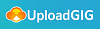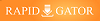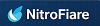# Trigonometry - The Unit Circle, Angles & Right Triangles

#### Category: Tutorial

Posted on 2021-12-26, by voska89.

DescriptionMP4 | Video: h264, 1280x720 | Audio: AAC, 44.1 KHz
Language: English | Size: 1.83 GB | Duration: 19h 16m
Analytical Trig, Verifying Trigonometric Identities, Double Angle Formulas, Inverse Trigonometric Functions & More.

What you'll learn
Students should have a solid understanding of trigonometry if they work through all of the example problems.
Description
This online trigonometry course is for high school and college students who are taking trig and may need help with their homework or simply to pass a test. Here is a list of topics contained in this video.
1. Introduction to Angles - Drawing In Standard Position
3. Circular Arc Length, Linear Speed and Angular Speed
4. Coterminal Angles and Reference Angles
5. The Six Trigonometric Functions and The Unit Circle
6. Finding Exact Values of Trigonometric Functions - Sine, Cosine, and Tangent
7. Even and Odd Trigonometric Functions, Reciprocal Identities, and Quotient Identities
8. Fundamental Pythagorean Identities With Sine and Cosine
9. Right Triangle Trigonometry - SOHCAHTOA and Pythagorean Theorem
10. 30-60-90 and 45-45-90 Right Triangles
11. Angle of Elevation and Angle of Depression Word Problems
12. Trigonometric Functions of Any Angle - Using Reference Angles To Find The Exact Value
13. Evaluating Trigonometric Functions of Quadrantal Angles
14. The Signs of Trigonometric Functions In Quadrants 1 to 4
15. Graphing Sine and Cosine Functions - Identifying The Amplitude, Period, Phase Shift, and Vertical Shift
16. The Graphs and Secant and Cosecant Functions - Domain and Range
17. Graphing Tangent and Cotangent Functions - Identifying The Vertical Asymptote
18. Inverse Trigonometric Functions
19. Inverse Sine Function From Restricted Sine Graph - One to One Function & Horizontal Line Test
20. Finding The Exact Value of an Inverse Sine and Cosine Function
21. Graphing The Inverse Sine, Cosine, and Tangent Function
22. Domain and Range of Inverse Trig Functions
23. Evaluating a Composite Trigonometric Expression With Inverse Functions
24. Applications of Trigonometric Functions - Solving Two Right Triangle Systems
25. Introduction to Bearings - Word Problems
26. Verifying Trigonometric Identities - Changing Sines to Cosines and Factoring
27. Sum and Difference Formulas of Sine, Cosine, and Tangent - Alpha and Beta Angles
28. Finding Exact Values With Sum and Difference Formulas
29. Verifying Trig Identities With Sum and Difference Formulas
30. Using Double Angle Formulas For Sine, Cosine, and Tangent To Find Exact Values
31. Simplifying Trigonometric Expressions With Power Reducing Formulas
32. Half Angle Formulas and Identities
33. Verifying Trigonometric Identities With Double Angle Formulas
34. Inverse Trigonometric Functions With Double Angle Formulas and Half Angle Identities
Who this course is for
This course is for high school and college students who wish to do well in Trigonometry.
https://www.udemy.com/course/trigonometry-the-unit-circle-angles-right-trianglesMore Course Expensive Download Click Here : https://www.ebookee.com/user/voska89https://rapidgator.net/file/ce74a3a1b9c7f98d54061ba273a7d45a/lf9i0.T..T.U.C.A..R.T.rar.html7137 dl's @ 2545 KB/s
5443 dl's @ 2925 KB/s
5917 dl's @ 2881 KB/s

Search More...
Trigonometry - The Unit Circle, Angles & Right TrianglesRelated Books